# Write an equation in standard form using only integers for theline described. Use the smallest...

Write an equation in standard form using only integers for the line described. Use the smallest possible positive integer coefficient for x. Then make a sketch. The line perpendicular to x−3y=7 and containing (−3,4).

The equation in standard form using only integers is ______? (Type an equation. Simplify your answer.)

SOLUTION :

Line : x - 3y = 7

=> y = x/3 - 7/3

Its slope = 1/3

Slope of line perpendicular to it = -1 / (1/3) = - 3 .

So, equation of perpendicular line is :

y = - 3x + c

Since point (-3, 4) is on this line,

4 = - 3(-3) + c

=> c = 4 - 9 = - 5

So, equation of perpendicular line is :

y = - 3x - 5

In standard form equation is :

3x + y = - 5 (ANSWER).

So,

x-intercept is (at y = 0) : x = - 5/3

y-intercept is (at x = 0) : y = - 5 .

Plot these points and draw a line through  these points. It is the graph of the perpendicular line.  Use demos.com to check. (ANSWER).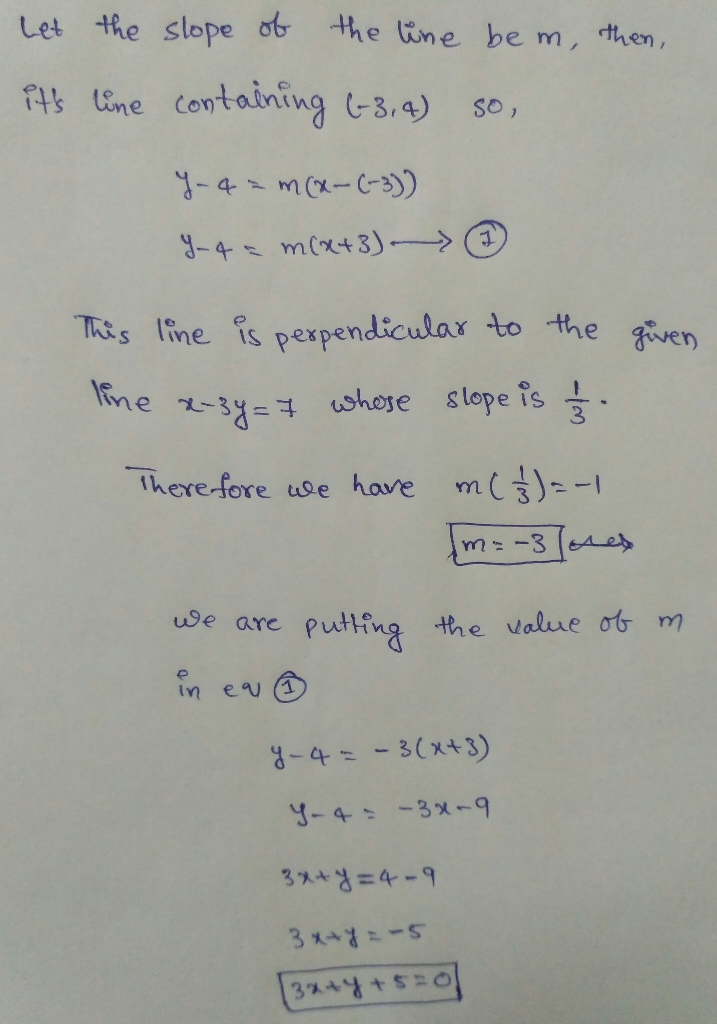#### Earn Coin

Coins can be redeemed for fabulous gifts.

Similar Homework Help Questions
• ### Write the equation in standard form using only integers and a positive coefficient for x

Write the equation in standard form using only integers and a positive coefficient for x. Match your result to the correct answer below. a..x + 3y = 6 b...x  3y = 2 c...x + 3y = 6 d...x  3y = 6

• ### 1) Write the equation y=1/3x - 2 in standard form using only integers and a positive coefficient for x

1) Write the equation y=1/3x - 2 in standard form using only integers and a positive coefficient for x. a) x+3y=6 b) x-3y=2 c)-x+3y=-6 d)x-3y=6 20 Which of the following equations represents a line l that goes through (-1,-3) and has a slope of 4? a)y=4x-3 b)y=4x+1 c)y=4x-1 d)y=4x+3 Please, can anyone help me with the above? thank you so much.

• ### a.)Write a balanced equation for the reaction described, using the smallest possible integer coefficients. When nitrogen...

a.)Write a balanced equation for the reaction described, using the smallest possible integer coefficients. When nitrogen monoxide reacts with oxygen, nitrogen dioxide is formed. _____________+____________-------------> _____________ b.) Write a balanced equation for the reaction described, using the smallest possible integer coefficients. Pure sodium nitrate(NaNO3) undergoes a chemical change to form, sodium nitrite(NaNO2) and oxygen. ______________ --------> ___________ + _____________ c.) Write a balanced equation for the reaction described, using the smallest possible integer coefficients. When aqueous solutions of potassium hydroxide...

• ### Use the given conditions to write an equation for the line in standard form. Passing through...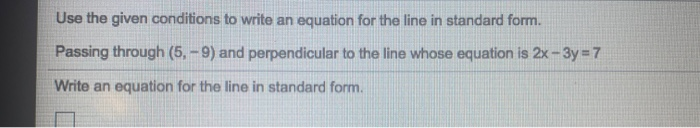Use the given conditions to write an equation for the line in standard form. Passing through (5.-9) and perpendicular to the line whose equation is 2x - 3y = 7 Write an equation for the line in standard form.

• ### Write a balanced equation for the decomposition reaction described, using the smallest possible integer coefficients. When...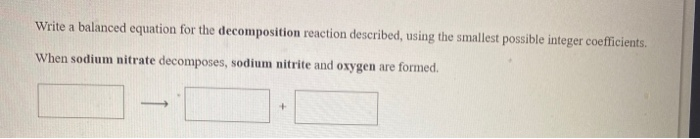Write a balanced equation for the decomposition reaction described, using the smallest possible integer coefficients. When sodium nitrate decomposes, sodium nitrite and oxygen are formed Write a balanced equation for the combination reaction described, using the smallest possible integer coefficients. When sulfur dioxide combines with oxygen, sulfur trioxide is formed.

• ### Use the given conditions to write an equation for the line in point-slope form and in...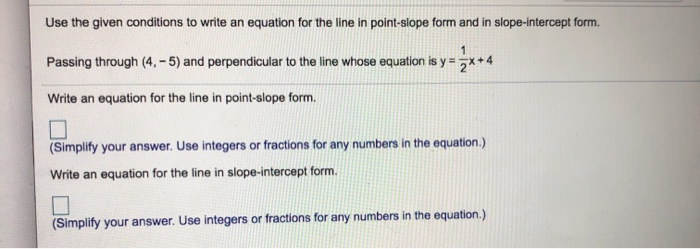Use the given conditions to write an equation for the line in point-slope form and in slope-intercept form. 1 Passing through (4. - 5) and perpendicular to the line whose equation is y= 3x+4 Write an equation for the line in point-slope form. (Simplify your answer. Use integers or fractions for any numbers in the equation.) Write an equation for the line in slope-intercept form. (Simplify your answer. Use integers or fractions for any numbers in the equation.)

• ### Write a balanced equation for the double-replacement precipitation reaction described, using the smallest possible integer coefficients....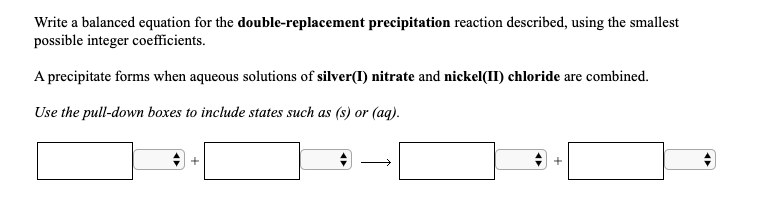Write a balanced equation for the double-replacement precipitation reaction described, using the smallest possible integer coefficients. A precipitate forms when aqueous solutions of silver(I) nitrate and nickel(II) chloride are combined. Use the pull-down boxes to include states such as (s) or (aq). Write a balanced equation for the double-replacement precipitation reaction described, using the smallest possible integer coefficients. A precipitate forms when aqueous solutions of silver(I) nitrate and nickel(II) chloride are combined. Use the pull-down boxes to include states such...

• ### Write a balanced equation for the double-replacement precipitation reaction described, using the smallest possible integer coefficients....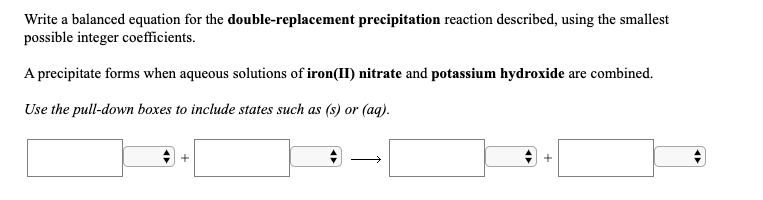Write a balanced equation for the double-replacement precipitation reaction described, using the smallest possible integer coefficients. A precipitate forms when aqueous solutions of iron(II) nitrate and potassium hydroxide are combined. Use the pull-down boxes to include states such as (s) or (aq). Write a balanced equation for the double-replacement precipitation reaction described, using the smallest possible integer coefficients. A precipitate forms when aqueous solutions of iron(II) nitrate and potassium hydroxide are combined. Use the pull-down boxes to include states such...

• ### using point-slope form write an equation, perpendicular to the line y-4=-2(x-3), containing the point (3,4)

using point-slope form write an equation, perpendicular to the line y-4=-2(x-3), containing the point (3,4)

• ### When y-1=-4/5(x-3) is written in standard form using positive integers, what is the smallest possible coeficcient of x

When y-1=-4/5(x-3) is written in standard form using positive integers, what is the smallest possible coeficcient of x?